# First-order exact differential equation

## Definition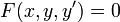$F(x,y,y') = 0$

is termed a first-order exact differential equation if we can find a function$R(x,y)$ such that: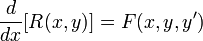$\frac{d}{dx}[R(x,y)] = F(x,y,y')$

where the differentiation symbol on the left stands for implicit differentiation, assuming$y$ an implicit function of$x$.

For a first-order exact differential equation, the general solution is: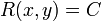$R(x,y) = C$

Note that this general solution is relational, though we may be able to convert it to a functional solution using algebraic manipulation.

If multiplying a first-order first-degree differential equation by a function makes it exact, this function that is multiplied is termed an integrating factor for the equation.

## Facts

Essentially, all methods of solving first-order first-degree differential equations involve converting them to exact differential equations:

Equation type of solution method How it essentially involves using an exact differential equation
separable differential equation Here, we attempt to write it as the implicit derivative of an additively separable function of$x$ and$y$. (don't confuse this with the fact that the derivative$dy/dx$ itself is multiplicatively separable). In the language of integrating factors,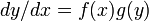$dy/dx = f(x)g(y)$ has$1/g(y)$ as its integrating factor.
first-order linear differential equation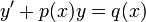$y' + p(x)y = q(x)$ Here, we use an integrating factor that is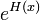$e^{H(x)}$ where$H$ is an antiderivative of$p$.
substitution method for solving differential equations This doesn't directly convert the differential equation to an exact form, but changes variables in a manner that it is easier to see the exact form. Note that by back substitution, we can convert the exact form in the substituted equation to an exact form for the original equation.

## Examples

We list here some examples that cannot be easily tackled using other methods.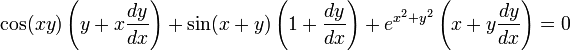$\cos(xy)\left(y + x\frac{dy}{dx}\right) + \sin(x + y)\left(1 + \frac{dy}{dx}\right) + e^{x^2 + y^2}\left(x + y \frac{dy}{dx}\right) = 0$

We note that:$\cos(xy)\left(y + x\frac{dy}{dx}\right) = \frac{d}{dx}(\sin(xy))$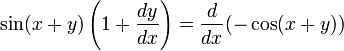$\sin(x + y)\left(1 + \frac{dy}{dx}\right) = \frac{d}{dx}(-\cos(x+ y))$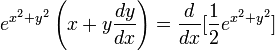$e^{x^2 + y^2}\left(x + y \frac{dy}{dx}\right) = \frac{d}{dx}[\frac{1}{2}e^{x^2 + y^2}]$

Thus, the overall differential equation is exact, and is of the form: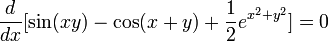$\frac{d}{dx}[\sin(xy) - \cos(x + y) + \frac{1}{2}e^{x^2 + y^2}] = 0$

Integrating, we get the following family of relational solutions: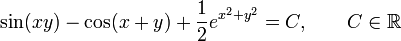$\sin(xy) - \cos(x + y) + \frac{1}{2}e^{x^2 + y^2} = C, \qquad C \in \R$